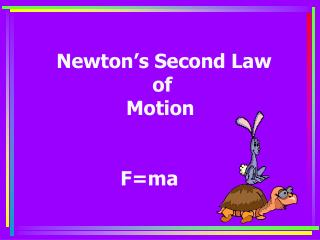DownloadDownload PresentationNewton’s Second Law of Motion F=ma

Newton’s Second Law of Motion F=ma

Télécharger la présentationNewton’s Second Law of Motion F=ma

- - - - - - - - - - - - - - - - - - - - - - - - - - - E N D - - - - - - - - - - - - - - - - - - - - - - - - - - -
Presentation Transcript

1. Newton’s Second Law of Motion F=ma

2. Why can a rabbit run faster than a turtle? It’s really quite simple. He can apply more force to his mass than I can.

3. Truck A Truck B Which of these trucks can accelerate the easiest? Why? GET OUTSIDE THE BOX Prove your answer with realistic numbers. Remember Force (F) and mass (m) ma F =

4. A F m a = 10,000 N 8,000 kg a = a = 1.26 m/sec2 F m B a = 10,000 N 2,000 kg a = 5 m/sec2 a = With the same amount of force applied to each, B will accelerate easier because it has less mass.

5. How does an ice skater use force? • The skater uses force to get started and to speed up. 2. The skater uses force to changedirection. • C. ALL OF THESE ARE

6. m = 0.5 kg F = 2 N A car rolls down a ramp and you measure a force of 2 newtons pulling the car down. The mass of the car is 0.5 kg. What is its acceleration? Solve for acceleration. What formula would you use to solve for Force (F)? F m a = F = m x a 2 N 0.5 kg for mass (m)? a = _F_ a M = a = 4 m/sec2

7. BALANCED FORCES Balanced forces are always equal in size and opposite in direction. Balanced forces produce NO MOTION This man is pushing against a wall, the wall is pushing back against the man. Nothing moves so the forces are in equilibrium, (balanced)… NOTHING MOVES ! Notice that the arrows are the same size and pointing in opposite directions.

8. UNBALANCED FORCES With unbalanced forces the forces will be in opposite directions but the size of one force will be larger than the size of the other. UNBALANCED FORCES ALWAYS PROCDUCE MOTION ! As this man applies an unbalanced force to the dolly with the drinks, it moves because his force is more than the force of the object. (notice the arrows)

9. Analyze this force diagram What is the actual “force” applied by the dirt?(not a number) The weight of the dirt Opposite force applied by dirt Force applied by Dozer Rat. Direction of motion

10. We Need HELLLP! Analyze these force diagrams. Are you pulling ? The sum of all forces pulling in this direction The sum of all forces pulling in that direction Direction of motion

11. 3.2 WEIGHT, GRAVITY, & FRICTION In order to understand what weight is you must under- stand what gravity is. So….. Explain gravity Gravity is a force that pulls every mass towards every other mass. Gravity depends on mass, the greater the mass an object has, the greater its gravitational pull. For example, the earth has more mass than our moon, so the gravitational pull of earth is more than the moon. So… how does that relate to the weight of an object?

12. Let’s look at Mrs. Shelly weighing. How do these scales “weigh” us? The top platform of the scale is able to move and is attached by springs to the dial which are calibrated to show pounds of weight. Gravity pulls down on the mass of the person The floor pushes up on the scales

13. Calculating weight Mass (kg) Fw = mg Weight force (N) Acceleration of gravity (9.8 m/sec2) The correct unit for weight force is the newton (N). What is your metric weight? (reminder 1 kg=~2.2 lb.) Divide your weight by 2.2 lbs to convert it to mass, then multiply by 9.8m/sec2. This will give your wt. in newtons.

14. FRICTION Friction is a term used to describe forces that result from the relative motion between objects. Simply defined, friction is a force that opposes motion. Friction is caused by two surfaces rubbing against one another. The amount of friction depends on the smoothness or roughness of the surfaces. This is called texture.

15. REVIEW Newton’s 2nd Law of Motion is a mathematical equation which explains the relationship between force, mass, and acceleration. Acceleration (m/sec2) Force (Newtons, N) F = ma Mass (kilogams, kg)